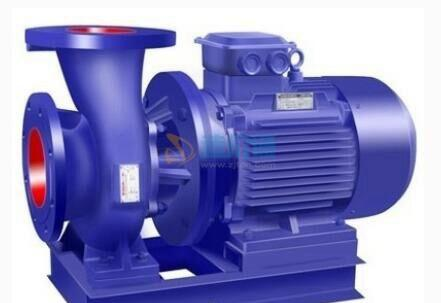[切换站点]

## 材料分类

IS型 IS50-32-250 (配二级电机) 11KW• IS型 IS50-32-250 (配二级电机) 11KW

2019-05

IS型 IS65-50-160A (配二级电机) 4KW• IS型 IS65-50-160A (配二级电机) 4KW

2019-05

IS型 IS65-50-160B (配二级电机) 3KW• IS型 IS65-50-160B (配二级电机) 3KW

2019-05

GDD125-32A Q=150m3/h H=28m N=18.5kW• GDD125-32A Q=150m3/h H=28m N=18.5kW

2019-05

GDD125-32A Q=150m3/h H=28m N=18.5kW• GDD125-32A Q=150m3/h H=28m N=18.5kW
13% 泉州市丰泽华茂锅炉配件贸易有限公司
2019-05

GDD125-50 Q=160m3/h H=50m N=37kW• GDD125-50 Q=160m3/h H=50m N=37kW

2019-05

GDD150-32A Q=187m3/h H=28m N=22kW• GDD150-32A Q=187m3/h H=28m N=22kW

2019-05

GDD200-24 Q=300m3/h H=24m N=30kW• GDD200-24 Q=300m3/h H=24m N=30kW

2019-05

GDD200-48B Q=250m3/h H=37m N=37kW• GDD200-48B Q=250m3/h H=37m N=37kW

2019-05

GDD250-20 Q=400m3/h H=20m N=30kW• GDD250-20 Q=400m3/h H=20m N=30kW

2019-05

GDD250-32A Q=374m3/h H=28m N=25kW• GDD250-32A Q=374m3/h H=28m N=25kW

2019-05

GDD250-50B Q=340m3/h H=38m N=55kW• GDD250-50B Q=340m3/h H=38m N=55kW

2019-05

IS100-65-200 Q=100m3/h H=50m N=22kW• IS100-65-200 Q=100m3/h H=50m N=22kW

2019-05

IS100-65-200A Q=93.5m3/h H=44m N=18.5kW• IS100-65-200A Q=93.5m3/h H=44m N=18.5kW

2019-05

IS100-65-250B Q=79m3/h H=60m N=22kW• IS100-65-250B Q=79m3/h H=60m N=22kW

2019-05

IS100-80-125 Q=100m3/h H=20m N=11kW• IS100-80-125 Q=100m3/h H=20m N=11kW

2019-05

IS型 IS50-32-250B (配二级电机) 5.5KW• IS型 IS50-32-250B (配二级电机) 5.5KW
13% 泉州市丰泽华茂锅炉配件贸易有限公司
2019-05

IS型 IS65-40-200 (配二级电机) 7.5KW• IS型 IS65-40-200 (配二级电机) 7.5KW
13% 泉州市丰泽华茂锅炉配件贸易有限公司
2019-05

IS型 IS65-40-200B (配二级电机) 4KW• IS型 IS65-40-200B (配二级电机) 4KW
13% 泉州市丰泽华茂锅炉配件贸易有限公司
2019-05

IS型 IS65-50-160A (配二级电机) 4KW• IS型 IS65-50-160A (配二级电机) 4KW
13% 泉州市丰泽华茂锅炉配件贸易有限公司
2019-05
1 2 3 4 5 6 7 下一页 跳到 GO